# 基于OpenCV 的车牌识别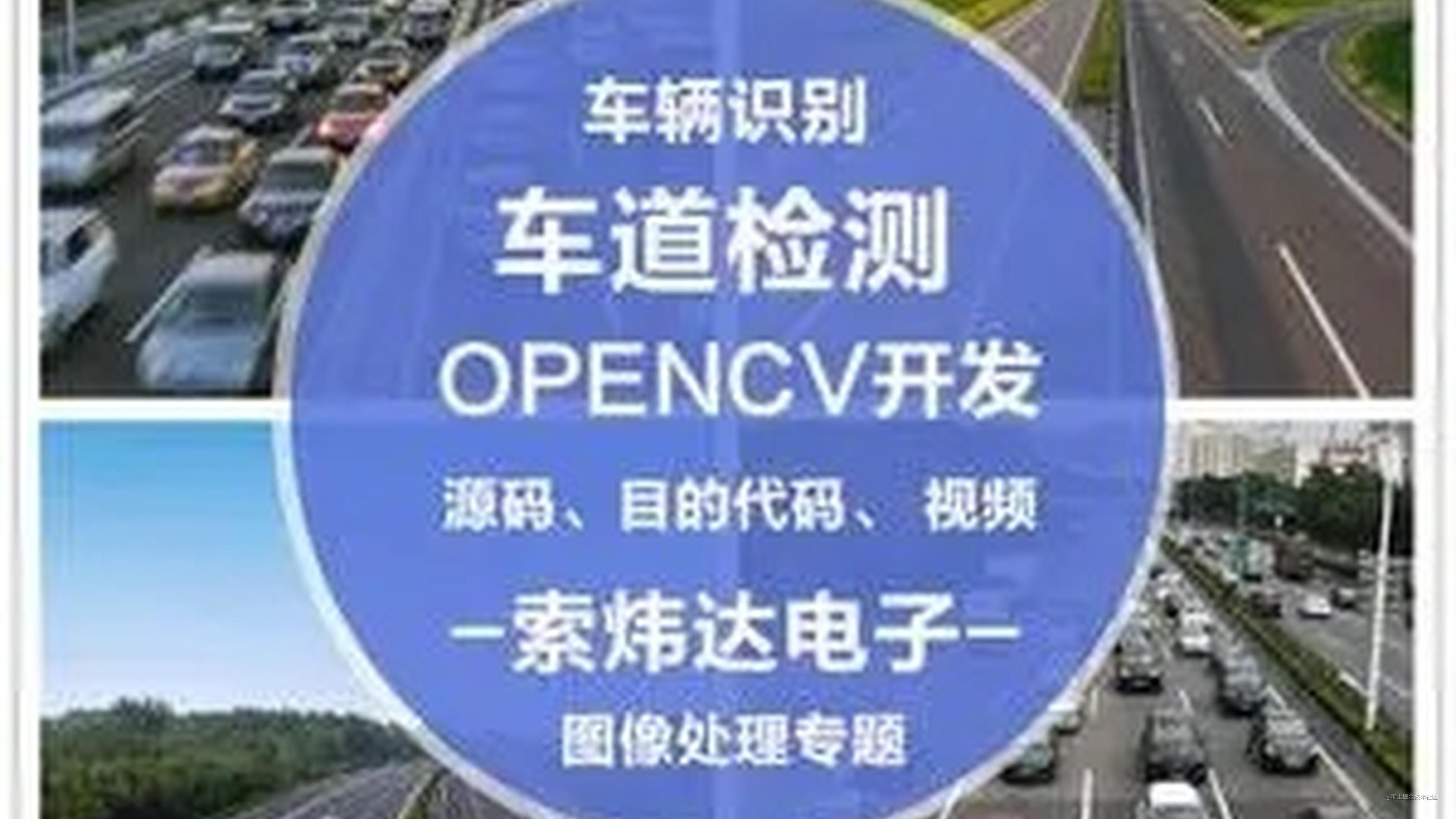1.车牌检测： 第一步是从汽车上检测车牌所在位置。我们将使用OpenCV中矩形的轮廓检测来寻找车牌。如果我们知道车牌的确切尺寸，颜色和大致位置，则可以提高准确性。通常，也会将根据摄像机的位置和该特定国家/地区所使用的车牌类型来训练检测算法。但是图像可能并没有汽车的存在，在这种情况下我们将先进行汽车的，然后是车牌。

2.字符分割： 检测到车牌后，我们必须将其裁剪并保存为新图像。同样，这可以使用OpenCV来完成。

3. 字符识别： 现在，我们在上一步中获得的新图像肯定可以写上一些字符（数字/字母）。因此，我们可以对其执行OCR（光学字符识别）以检测数字。

1.车牌检测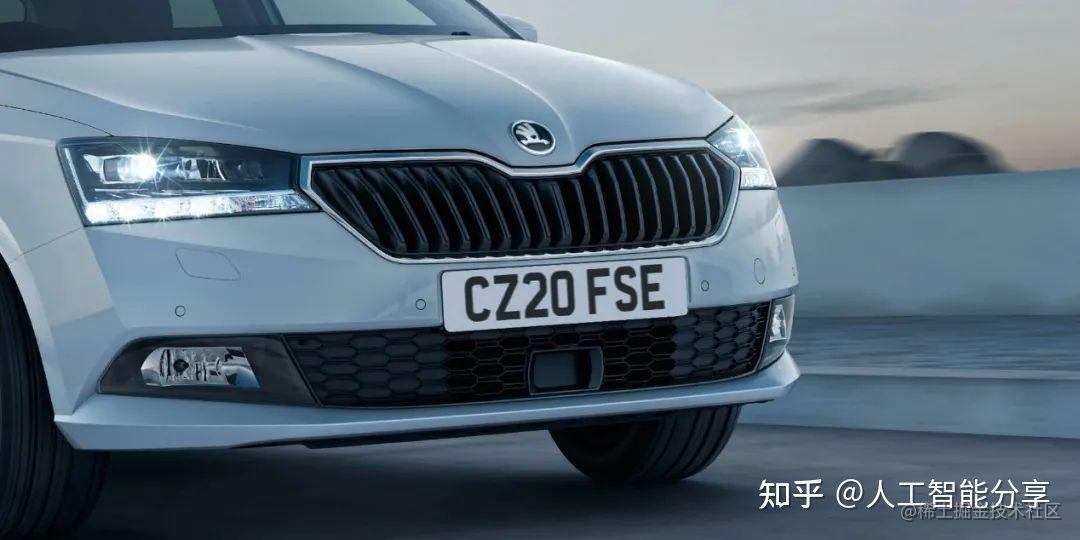``````img = cv2.resize(img, (620,480) )gray = cv2.cvtColor(img, cv2.COLOR_BGR2GRAY) #convert to grey scale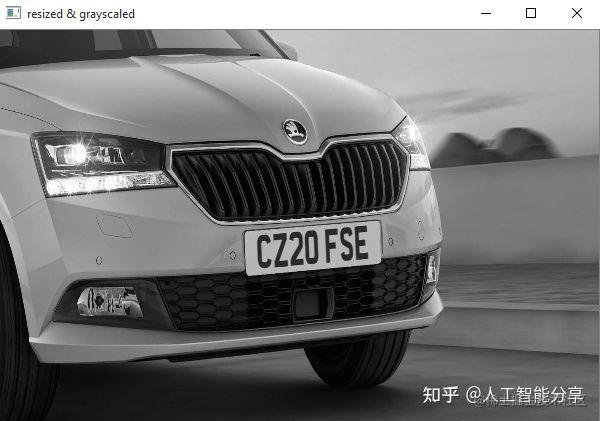``````gray = cv2.bilateralFilter(gray, 13, 15, 15)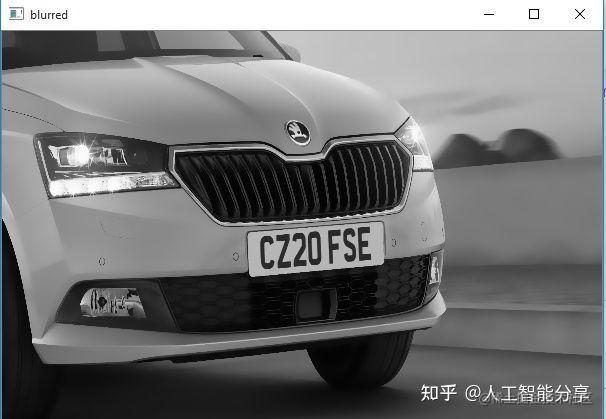``````edged = cv2.Canny(gray, 30, 200) #Perform Edge detection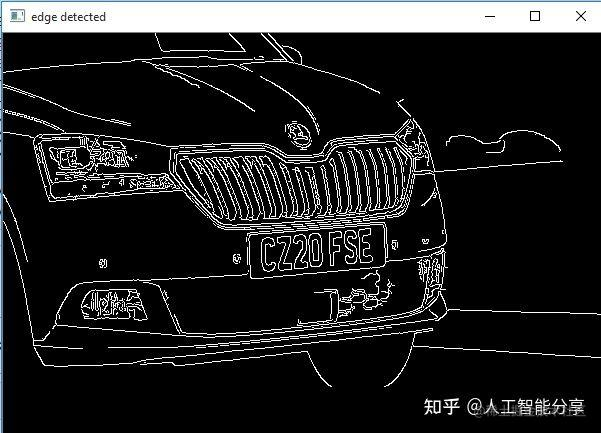``````contours=cv2.findContours(edged.copy(),cv2.RETR_TREE,

``````for c in cnts:    # approximate the contour    peri = cv2.arcLength(c, True)    approx = cv2.appr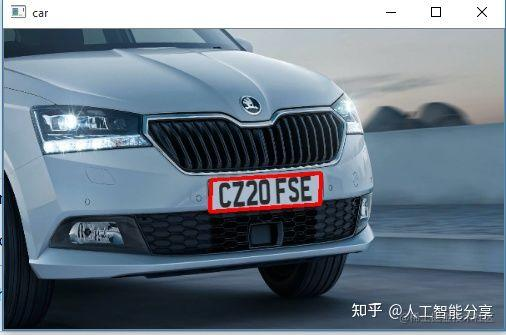``````# Masking the part other than the number platemask = np.zeros(gray.shape,np.uint8)new_image = cv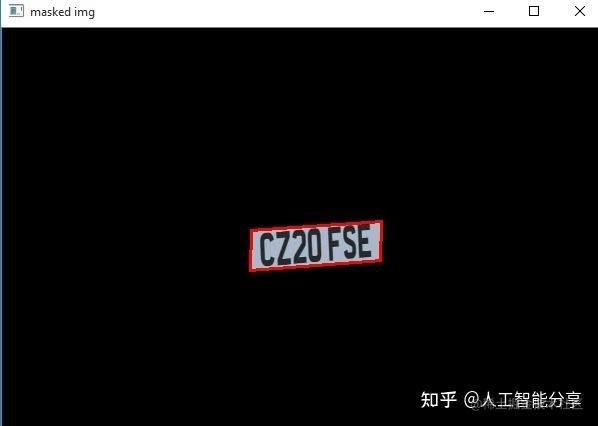2.字符分割

``````# Now crop(x, y) = np.where(mask == 255)(topx, topy) = (np.min(x), np.min(y))(bottomx, bottomy) =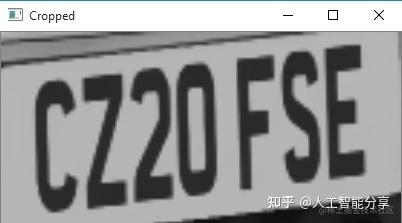3.字符识别

``````#Read the number platetext = pytesseract.image_to_string(Cropped, config='--psm 11')print("Detec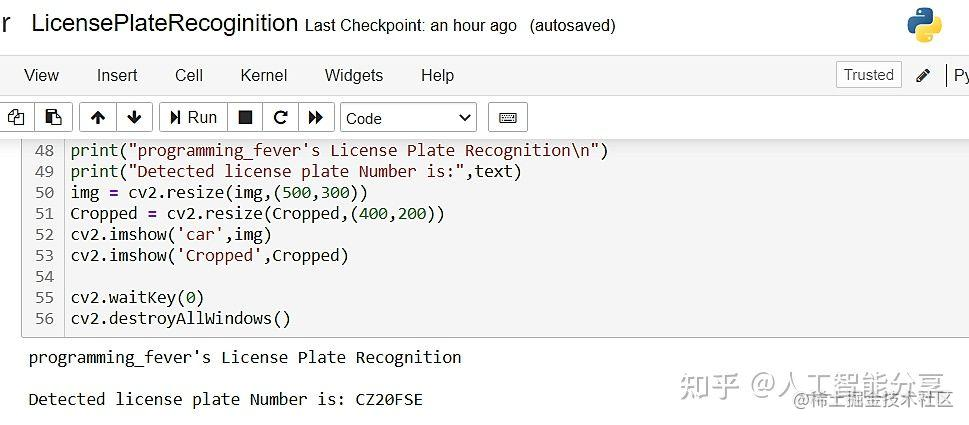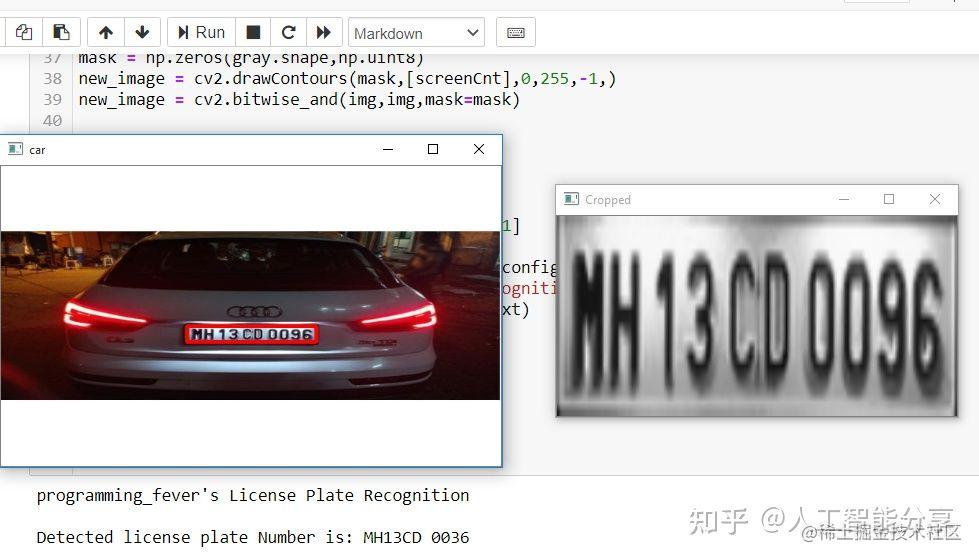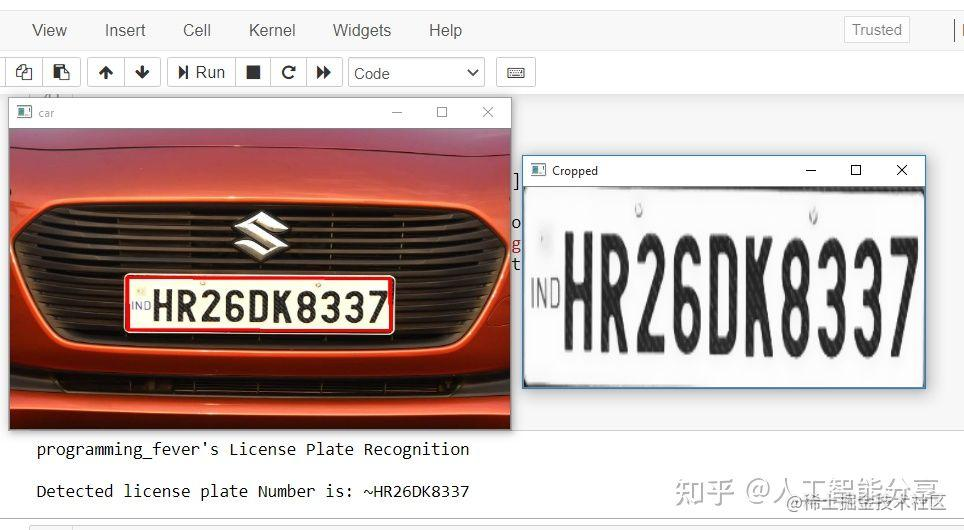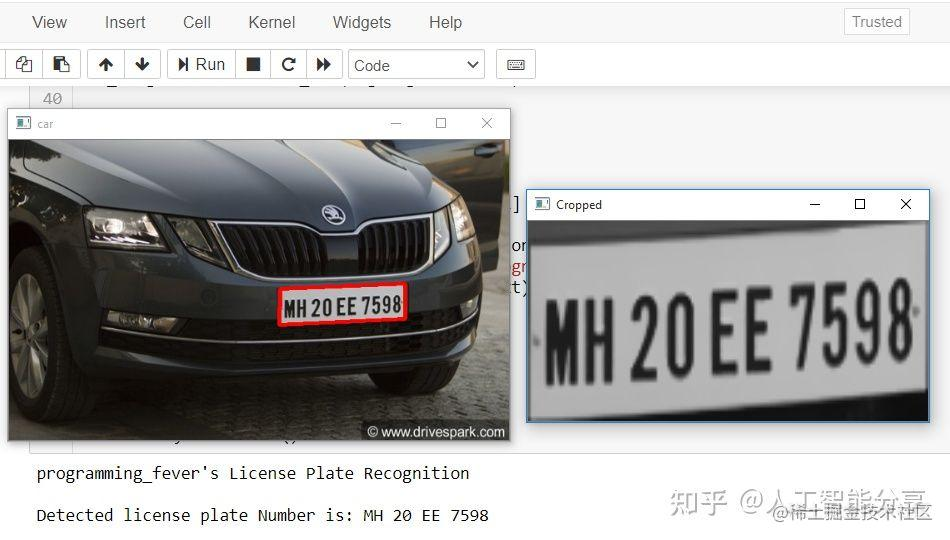``````#@programming_feverimport cv2import imutilsimport numpy as npimport pytesseractpytesseract.pytesseract.tesseract_cmd = r'C:\Program Files (x86)\Tesseract-OCR\tesseract.exe'
img = cv2.imread('D://skoda1.jpg',cv2.IMREAD_COLOR)img = cv2.resize(img, (600,400) )
gray = cv2.cvtColor(img, cv2.COLOR_BGR2GRAY) gray = cv2.bilateralFilter(gray, 13, 15, 15)
edged = cv2.Canny(gray, 30, 200) contours = cv2.findContours(edged.copy(), cv2.RETR_TREE, cv2.CHAIN_APPROX_SIMPLE)contours = imutils.grab_contours(contours)contours = sorted(contours, key = cv2.contourArea, reverse = True)[:10]screenCnt = None
for c in contours:        peri = cv2.arcLength(c, True)    approx = cv2.approxPolyDP(c, 0.018 * peri, True)     if len(approx) == 4:        screenCnt = approx        break
if screenCnt is None:    detected = 0    print ("No contour detected")else:     detected = 1
if detected == 1:    cv2.drawContours(img, [screenCnt], -1, (0, 0, 255), 3)
(x, y) = np.where(mask == 255)(topx, topy) = (np.min(x), np.min(y))(bottomx, bottomy) = (np.max(x), np.max(y))Cropped = gray[topx:bottomx+1, topy:bottomy+1]
text = pytesseract.image_to_string(Cropped, config='--psm 11')print("programming_fever's License Plate Recognition\n")print("Detected license plate Number is:",text)img = cv2.resize(img,(500,300))Cropped = cv2.resize(Cropped,(400,200))cv2.imshow('car',img)cv2.imshow('Cropped',Cropped)
cv2.waitKey(0)cv2.destroyAllWindows()